# AP Chemistry : Atomic Structure and Electron Configuration

## Example Questions

← Previous 1 3

### Example Question #1 : Atomic Structure And Electron Configuration

If an electron falls from the energy level of n = 5 to the ground state of n = 1, what is most likely to occur?

The electron changes its spin direction
A neutron is transformed into a proton
A photon is absorbed
A photon is emitted
The electron gains velocity
Correct answer: A photon is emitted
Explanation:

There is not enough information given in the question stem to determine if the velocity of the electron is changed. We can definitively determine, however, the electron will lose energy and a photon will be emitted.

### Example Question #1 : Atomic Structure And Electron Configuration

Which is the most stable electron configuration of Fe (II) ion?

1s2 2s2 2p6 3s2 3p6
1s2 2s2 2p6 3s2 3p6 3d6
1s2 2s2 2p6 3s2 3p6 4s2 3d4
1s2 2s2 2p6 3s2 3p4 4s2
1s2 2s2 2p6 3s2 3p6 4s2 3d6
Correct answer: 1s2 2s2 2p6 3s2 3p6 3d6
Explanation:

A fully filled orbital is generally more stable than a half-filled orbital. The splitting of the energy levels is also dependent on the geometry of the compound that one is analyzing. The 4s electrons are of higher energy than the 3d electrons, thus are lost first, leaving half filled d orbitals, which is the most stable configuration.

### Example Question #1 : Atomic Structure And Electron Configuration

Which atomic subshell fills with electrons first: 3d or 4s?

4s

3d

Both will fill simultaneously

Not enough information given to answer

4s

Explanation:

For the 3d subshell, n (principal quantum number) = 3 and l (azimuthal quantum number) = 2, so n + l = 5. For the 4s subshell, n = 4 and l = 0, so n+l = 4. From this information, we can see that the 4s subshell has lower energy and will fill with electrons first.

### Example Question #1 : Atomic Structure And Electron Configuration

Which is the correct orbital notation for Copper?

1s2 2s2 2p6 3s2 3p6 4s2 3d9

1s2 2s2 2p6 3s2 3p6 3d10

1s2 2s2 2p6 3s2 3p6 4s1 3d10

1s2 2s2 2p6 3s2 3p6 4s2 3d10

1s2 2s2 2p6 3s2 3p6 4s1 3d10

Explanation:

The elements in copper's group will fill the d orbital at the expense of having a half filled s orbital. This is because this configuration is more stable than a full s orbital and d orbital with 9/10 spots filled.

### Example Question #1 : Atomic Structure And Electron Configuration

What is the electron configuration for Chromium?

[Ar] 3d4 4s2

[Ar] 3d6

[Ar] 3p6

[Ar] 3d5 4s1

[Ar] 3d5 4s1

Explanation:

6 more electrons are present in chromium than argon. It's more stable for d orbitals to be half-filled. Thus, one electron will fill each of the 5 d orbitals.

### Example Question #1 : Atomic Structure And Electron Configuration

What is the element with the ground electron configuration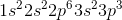?

Silicon

Sulfur

Aluminum

Phosphorous

Potassium

Phosphorous

Explanation:

Electron configurations can easily be figured out by using the periodic table. It is important to memorize the order that the orbitals become filled; then, you can simply follow the periodic table, adding one electron as you move from one element to the next. To make things easier, you can also remember that the first period ends with 1s, the second ends with 2p, and the third ends with 3p. It gets a little more complicated after that, but knowing those first few periods will let you work quicker.

When given an electronic configuration, you can also add the number of electrons to determine the element's atomic number.shows a total of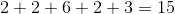electrons. Phosphorous corresponds to atomic number 15.

### Example Question #1 : Atomic Structure And Electron Configuration

Which element has a valence shell with five electrons?

Arsenic

Carbon

Bromine

Aluminum

Sulfur

Arsenic

Explanation:

To find the answer, we would write out the electron configuration for each given choice.

Arsenic has an electron configuration of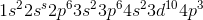, with the fourth shell representing the valence electrons. The valence includes both the 4s and 4p, so it has a total of 5 (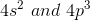).  We could also look at the periodic table and see that arsenic is in the same period as nitrogen and phosphorus, meaning it will have five valence electrons.

### Example Question #2 : Atomic Structure And Electron Configuration

How many valence orbitals contain one electron in a nitrogen atom?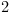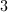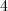Explanation:

Nitrogen is an atom that is beginning to have its p orbitals filled with electrons. According to Hund's rule, electrons will prefer an unoccupied orbital when it is at the same energy level as the other available orbitals. In nitrogen, the 2s orbital has been completely filled. Since there are three p orbitals available, the three remaining electrons will prefer to have their own unoccupied orbitals. As a result, the three p orbitals will each have one electron.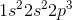1s ee

2s ee

2p e_ e_ e_

### Example Question #2 : Atomic Structure And Electron Configuration

Which element does not contain electrons in the d orbital?

Calcium

Strontium

Krypton

Gallium

Scandium

Calcium

Explanation:

The periodic table is designed to organize elements by their orbitals. Electrons only enter the d orbital in the fourth period (row) at the beginning of the transition metals. Scandium is the first element to have an electron enter the d orbital. Calcium is the last element on the table that has up to p orbital electrons, and empty d orbitals.

Calcium: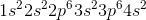Scandium: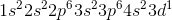### Example Question #2 : Atomic Structure And Electron Configuration

A ground state monovalent cation has the following electron configuration: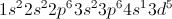What is the cation?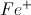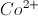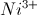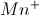Since this is a monovalent cation, the non-ionic electron configuration will be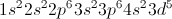(note that the electron was removed from the s shell and not the d shell, as this is a transition metal). This electron configuration corresponds to iron, so the cation must be.